﻿ 水库库容变化对蓟县地震台DSQ型水管倾斜仪观测的影响文章快速检索
 地震地磁观测与研究2019, Vol. 40Issue (2): 108-114  DOI: 10.3969/j.issn.1003-3246.2019.02.0150

### 引用本文Zhu Bingqing, Zhao Liming, Zhang Lei, et al. Effect of reservoir capacity change on observation of DSQ type water pipe inclinometer at Jixian Seismic Station[J]. Seismological and Geomagnetic Observation and Research, 2019, 40(2): 108-114. DOI: 10.3969/j.issn.1003-3246.2019.02.015.### 文章历史

1. 中国天津 300201 天津市地震局;
2. 中国北京 100045 中国地震台网中心;
3. 中国合肥 230026 中国科学技术大学

Effect of reservoir capacity change on observation of DSQ type water pipe inclinometer at Jixian Seismic Station
Zhu Bingqing 1, Zhao Liming 1, Zhang Lei 1, Yan Wei 2, Huang Jinshui 3, Li Enjian 1, Xu Cheng 1, Li Yingnan 1, Cao Jingquan 1
1. Tianjin Earthquake Agency, Tianjin 300201, China;
2. China Earthquake Networks Center, Beijing 100045, China;
3. University of Science and Technology of China, Hefei 230026, China
Abstract: Compared with the reservoir capacity and the DSQ-type water pipe observation data, it shows the that reservoir capacity change has little influence on the long cycle trend of the Jixian Seismic Station. There is no correlation between the change of storage capacity and the observation data of the water pipe instrument. According to the three-dimensional irregular shape load model of the near field, the theoretical value of the change of the capacity of the reservoir during three times storage and three times discharge process of the Yuqiao reservoir vs the observation of the DSQ type water pipe instrument of Jixian Seismic Station is calculated. The results show that the slope of the water pipe caused by the change of the reservoir capacity is much smaller than the actual observation data variation. The storage capacity change is not enough to cause significant interference to the water pipe tilt observation. The analysis results can provide a reference for anomaly determination of observation data.
Key words: change of storage capacity    load calculation    ground tilt
0 引言

1 水库与观测山洞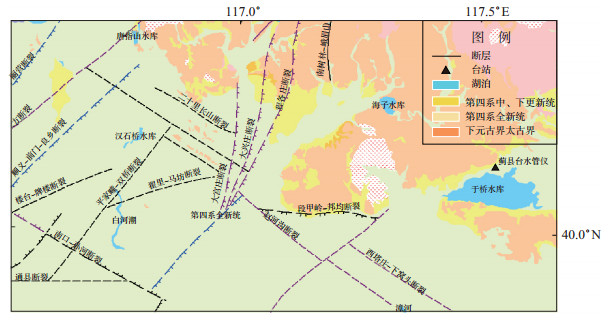图 1 于桥水库周边地质构造分布 Fig.1 Distribution of geological structure around Yuqiao reservoir

2 近场三维不规则形状荷载理论模型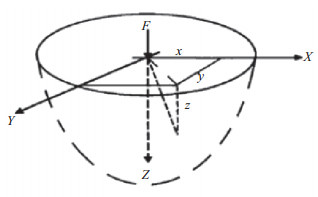图 2 半无限空间受垂向集中力作用示意 Fig.2 Schematic of vertical concentrated force in semi infinite space

 $W=\frac{P(1+\mu)}{2 \pi E}\left[\frac{Z^{2}}{R^{3}}+(2-\mu) \frac{1}{R}\right]$ (1)

 $T_{x}=\frac{\partial w}{\partial x}=\left[-3 \frac{P(1+\mu)}{2 \pi E} z^{2} R^{-4}-\frac{P(1+\mu)}{2 \pi E}(1-2 \mu) R^{-2}\right] R^{-1} x$ (2)
 $T_{y}=\frac{\partial w}{\partial y}=\left[-3 \frac{P(1+\mu)}{2 \pi E} z^{2} R^{-4}-\frac{P(1+\mu)}{2 \pi E}(1-2 \mu) R^{-2}\right] R^{-1} y$ (3)

 $\varphi_{x}=\arctan \left(T_{x}\right) \quad \varphi_{y}=\arctan \left(T_{y}\right)$ (4)

3 数据模拟计算

3.1 数据选取

3.2 模拟计算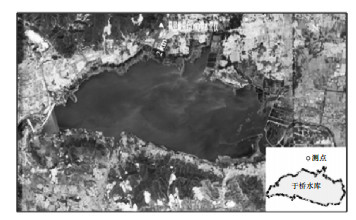图 3 仪器与于桥水库相对位置 Fig.3 Relative position of the instrument and Yuqiao reservoir表 1 库容变化对水管仪观测干扰的理论模拟值 Tab.1 Theoretical simulation value of disturbance observed by water pipe due to change of reservoir capacity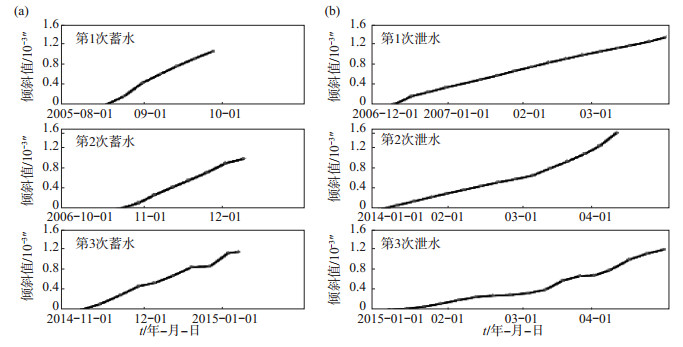图 4 水管仪NS向受于桥水库干扰模拟曲线 (a)水库蓄水时段；(b)水库泄水时段 Fig.4 Simulated curve of NS direction of water pipe inclinometer affected by the disturbance of reservoir
4 库容变化与观测值对比以及模拟结果分析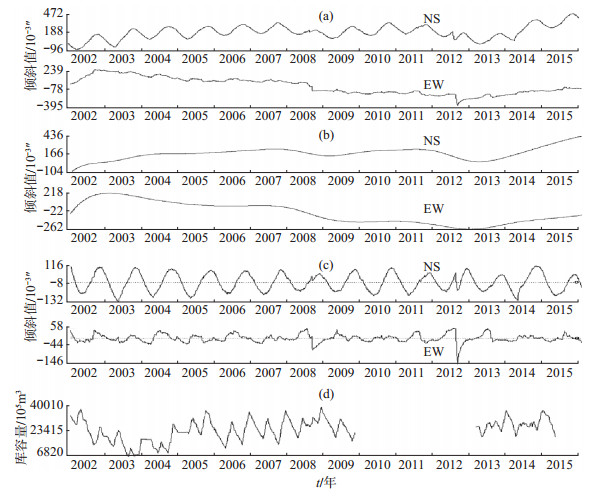图 5 蓟县台水管仪2002—2015年日均值时序曲线 (a)水管仪原始记录；(b)水管仪记录一般多项式分段拟合；(c)水管仪记录去趋势处理；(d)水库库容变化 Fig.5 Daily mean time series curves of water pipe inclinometer from 2002 to 2015 at Jixian Seismic Station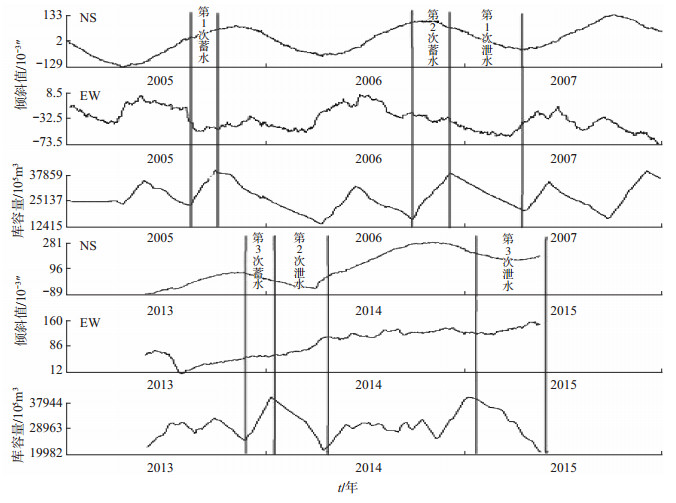图 6 蓟县台水管仪观测值与库容量对比曲线 Fig.6 Comparison of observational value and reservoir capacity at Jixian Seismic Station表 2 水库蓄水、泄水时段水管仪观测值和理论计算值对比 Tab.2 Comparisons between the observed and theoretical values of water pipe inclinometer during reservoir impoundment and discharge
5 结论

 李正媛, 陈鹏, 林穗平, 陈志遥. 川滇强震震源区形变潮汐短临变化特征[J]. 大地测量与地球动力学, 2003(2): 55-60. 裴晓峰. 抽水对蓟县台形变观测的干扰机理研究[J]. 地震地磁观测与研究, 1995, 16(4): 75-80. 孙强, 李继业, 李希文, 吴海波, 王强. 新城水库对延寿倾斜观测的影响评价[J]. 防灾科技学院学报, 2012, 14(1): 29-34. DOI:10.3969/j.issn.1673-8047.2012.01.006 汪翠枝, 陈嵩, 田山, 李恩建. 蓟县台地形变观测资料映震效能研究[J]. 地震, 2009, 29(3): 99-108. DOI:10.3969/j.issn.1000-3274.2009.03.013 闫伟, 牛安福, 陈国琴. 不规则载荷影响地表近场垂向位移和倾斜观测的定量计算[J]. 中国地震, 2015, 31(2): 297-307. DOI:10.3969/j.issn.1001-4683.2015.02.014 张磊, 邵永新, 曹井泉, 李恩建, 金大利. 蓟县台水管倾斜仪NS向加速北倾异常分析[J]. 大地测量与地球动力学, 2016, 36(4): 372-376. 张燕, 吕品姬, 柳嘉. 长江三峡水库蓄水对断层活动的影响[J]. 武汉大学学报(信息科学版), 2017, 42(10): 1497-1500.